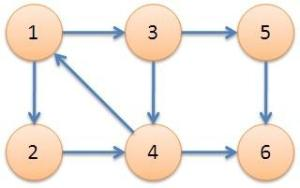# Tarjan算法 详解+心得

Tarjan算法是由Robert Tarjan（罗伯特·塔扬，不知有几位大神读对过这个名字） 发明的求有向图中强连通分量的算法。

预备知识：有向图，强连通。

有向图：由有向边的构成的图。需要注意的是这是Tarjan算法的前提和条件。

强连通：如果两个顶点可以相互通达，则称两个顶点 强连通(strongly connected)。如果有向图G的每两个顶点都 强连通，称G是一个强连通图。非 强连通图有向图的极大强连通子图，称为强连通分量(strongly connected components)。

For example:在这个有向图中1、2、3、4四个点可以互相到达，就称这四个点组成的子图为强连通分量。且这四个点两两强连通。

然后就可以开始学习神奇的Tarjan算法了！

Tarjan算法是用来求强连通分量的，它是一种基于DFS（深度优先搜索）的算法，每个强连通分量为搜索树中的一棵子树。并且运用了数据结构栈。

在介绍详细原理前，先引入两个非常重要的数组：dfn[ ] 与 low[ ]

dfn[ ]：就是一个时间戳（被搜到的次序），一旦某个点被DFS到后，这个时间戳就不再改变（且每个点只有唯一的时间戳）。所以常根据dfn的值来判断是否需要进行进一步的深搜。

low[ ]：该子树中，且仍在栈中的最小时间戳，像是确立了一个关系，low[ ]相等的点在同一强连通分量中。

注意初始化时 dfn[ ] = low[ ] = ++cnt.

算法思路：

首先这个图不一定是一个连通图，所以跑Tarjan时要枚举每个点，若dfn[ ] == 0，进行深搜。

然后对于搜到的点寻找与其有边相连的点，判断这些点是否已经被搜索过，若没有，则进行搜索。若该点已经入栈，说明形成了环，则更新low.

在不断深搜的过程中如果没有路可走了（出边遍历完了），那么就进行回溯，回溯时不断比较low[ ]，去最小的low值。如果dfn[x]==low[x]则x可以看作是某一强连通分量子树的根，也说明找到了一个强连通分量，然后对栈进行弹出操作，直到x被弹出。

先来一波局部代码加深一下理解：

void tarjan(int now)
{
dfn[now]=low[now]=++cnt;  //初始化
stack[++t]=now;　　　　　　 //入栈操作
v[now]=1;　　　　　　　　    //v[]代表该点是否已入栈
for(int i=f[now];i!=-1;i=e[i].next)  //邻接表存图
if(!dfn[e[i].v]) 　　　　　　　　　 //判断该点是否被搜索过
{
tarjan(e[i].v);
low[now]=min(low[now],low[e[i].v]); //回溯时更新low[ ]，取最小值
}
else if(v[e[i].v])
low[now]=min(low[now],dfn[e[i].v]); //一旦遇到已入栈的点，就将该点作为连通量的根
//这里用dfn[e[i].v]更新的原因是：这个点可能
//已经在另一个强连通分量中了但暂时尚未出栈，所
//以now不一定能到达low[e[i].v]但一定能到达
//dfn[e[i].v].
if(dfn[now]==low[now])
{
int cur;
do
{
cur=stack[t--];
v[cur]=false;                       //不要忘记出栈
}while(now!=cur);
}
}6无出度，之后判断 dfn［6］＝＝low［6］

low［2］＝1；

low［1］还是 1

。。。。。。。。。

End

#include<iostream>  //输出所有强连通分量
#include<cstdio>
#include<cstring>
#include<algorithm>
using namespace std;

int n,m,x,y,top=0,cnt=0,t,col;
int ans1=-1,ans2=-1,ans3=-1;
int d;
int a;
int c;
int f;
int dfn;
int low;
int stack;

bool v;

struct edge{
int u;
int v;
int w;
int next;
}e;

{
++top;
e[top].u=u;
e[top].v=v;
e[top].w=w;
e[top].next=f[u];
f[u]=top;
}

{
int x=0;
int k=1;
char c=getchar();
while(c>'9'||c<'0')
{
if(c=='-') k=-1;
c=getchar();
}
while(c>='0'&&c<='9')
x=x*10+c-'0',
c=getchar();
return x*k;
}

void tarjan(int now)
{
dfn[now]=low[now]=++cnt;
stack[++t]=now;
v[now]=1;
for(int i=f[now];i!=-1;i=e[i].next)
if(!dfn[e[i].v])
{
tarjan(e[i].v);
low[now]=min(low[now],low[e[i].v]);
}
else if(v[e[i].v])
low[now]=min(low[now],dfn[e[i].v]);
int cur;
if(dfn[now]==low[now])
{
do
{
cur=stack[t--];
v[cur]=false;
printf("%d ",cur);
}while(now!=cur);
printf("\n");
}
}

int main()
{
memset(f,-1,sizeof f);
for(int i=1;i<=n;++i)
for(int i=1;i<=m;++i)
{
}